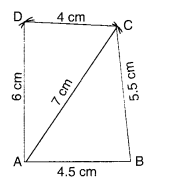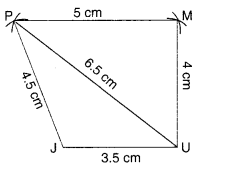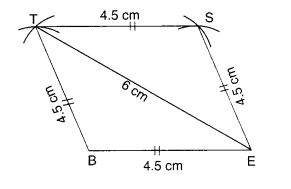NCERT Solutions for Class 8 Maths Chapter 4 Practical Geometry Ex 4.1 are part of NCERT Solutions for Class 8 Maths. Here we have given NCERT Solutions for Class 8 Maths Chapter 4 Practical Geometry Ex 4.1.

 Board CBSE Textbook NCERT Class Class 8 Subject Maths Chapter Chapter 4 Chapter Name Practical Geometry Exercise Ex 4.1 Number of Questions Solved 1 Category NCERT Solutions

## NCERT Solutions for Class 8 Maths Chapter 4 Practical Geometry Ex 4.1

Question 1.
AB = 4.5 cm
BC = 5.5 cm
CD = 4 cm
AC = 7 cm

JU = 3.5 cm
UM = 4 cm
MP = 5 cm
PJ = 4.5 cm
PU 6.5 cm

(iii) Parallelogram MORE
OR = 6 cm
RE = 4.5 cm
EO = 7.5 cm

(iv) Rhombus BEST
BE = 4.5 cm
ET = 6 cm
Solution.
(i) Steps of Construction

1. Draw AB 4.5 cm
2. With A as centre and radius AC = 7 cm, draw an arc.3. With B as center and radius BC = 5.5 cm, draw another arc to intersect the arc drawn in step (2) at C.
4. With A as center and radius AD = 6 cm, draw an arc on the side of AC, opposite to that of B.
5. With C as center and radius CD = 4 cm, draw another arc to intersect the arc drawn in step (4) at D.
6. Join BC, CD, DA, and AC.

Then, ABCD is the required quadrilateral.

(ii) Steps of Construction

1. Draw JU = 3.5 cm
2. With J as center and radius JP = 4.5 cm, draw an arc.
3. With U as center and radius UP = 6.5 cm, draw another arc to intersect the arc drawn in step 2 at P.4. With U as center and radius UM = 4 cm, draw an arc on the side of PU opposite to that of J.
5. With P as center and radius PM = 5 cm, draw another arc to intersect the arc drawn in step 4 at M.
6. Join UM, MP, PJ, and UP.

Then, JUMP is the required quadrilateral.

(iii) Steps of Construction
[We know that in a parallelogram, opposite sides are equal in length.
∴ MO = ER = 4.5 cm and ME – OR = 6 cm]

1. Draw MO = 4.5 cm
2. With M as center and radius ME = 6 cm, draw an arc.3. With O as center and radius OE = 7.5 cm, draw an arc to intersect the arc drawn in step 2 at E.
4. With O as center and radius OR = 6 cm, draw an arc on the side of OE opposite to that of M.
5. With E as center and radius ER = 4.5 cm, draw another arc to intersect the arc drawn in step 4 at R.
6. Join OR, RE, EM, and EO.

Then, MORE is the required parallelogram.

(iv) Steps of Construction
[We know that in a rhombus, all the sides are equal in length.
∴ BE = ES = ST = TB = 4.5 cm]

1. Draw BE = 4.5 cm
2. With B as centre and radius BT = 4.5 cm, draw an arc.3. With E as center and radius
ET = 6 cm, draw another arc to intersect the arc drawn in step 2 at T.
4. With E as center and radius
ES = 4.5 cm, draw an arc on the side of ET opposite to that of B.
5. With T as center and radius
TS = 4.5 cm, draw another arc to
intersect the arc drawn in step 4 at S.
6. Join ES, ST, TB, and TE.

Then, BEST is the required rhombus.

We hope the NCERT Solutions for Class 8 Maths Chapter 4 Practical Geometry Ex 4.1 help you. If you have any query regarding NCERT Solutions for Class 8 Maths Chapter 4 Practical Geometry Ex 4.1, drop a comment below and we will get back to you at the earliest.